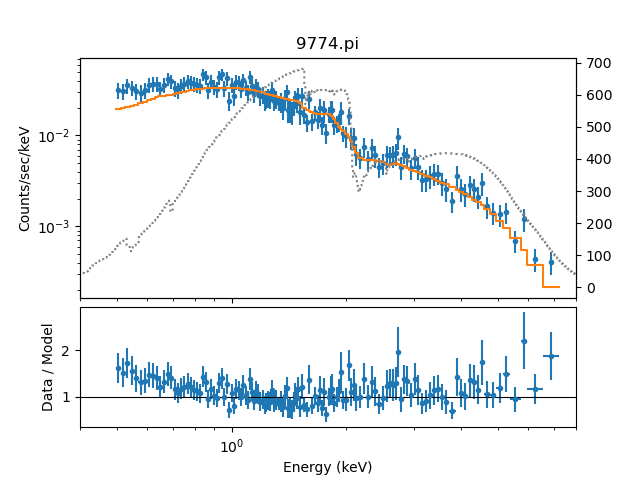# How can I include multiple datasets in the same plot?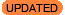New in CIAO 4.13

New in CIAO 4.13 is the ability to set the alpha transparency setting of the data with the alpha option.

In the following we assume you have two data sets loaded with ids of 1 (i.e. the default) and 2, that you have done the following

```sherpa> set_xlog()
sherpa> set_ylog()
```

and that fits have been made to both data sets.

If you wish to display multiple datasets in the same plot then you have to set the overplot argument to True; for example if you have two datasets with ids of 1 and 2 then:

```sherpa> plot_data(alpha=0.6)
sherpa> plot_data(2, alpha=0.6, overplot=True)
```

will display the two data sets in the same plot, with the second dataset drawn in orange. Note that the plot title is not changed when extra datasets are added.

### Overplotting different data sets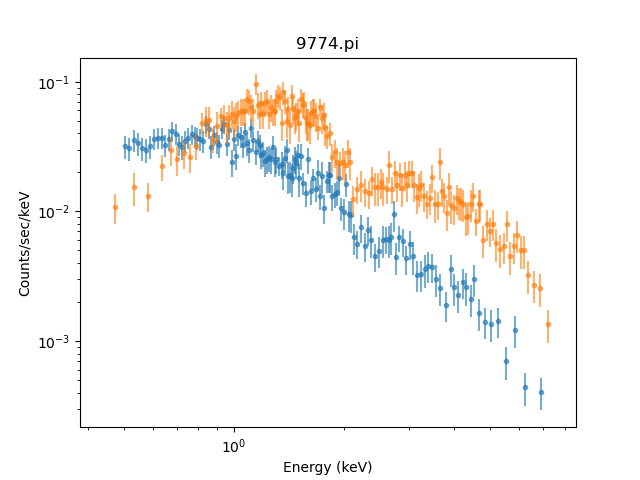### Overplotting different data sets

The two data set are drawn partially transparent; the alpha option, new in CIAO 4.13, goes from 0 (transparent) to 1 (fully opaque).

You can overplot data from the same data set; for instance if you want the residuals shown on the same graph as the data you could say

```sherpa> plot_fit(ylog=False)
sherpa> plot_resid(overplot=True, marker='s', markersize=3, alpha=0.5)
sherpa> ax = plt.hca()
sherpa> kwargs = {'horizontalalignment': 'right', 'transform': ax.transAxes}
sherpa> plt.text(0.9, 0.9, 'Data', color='blue', **kwargs)
sherpa> plt.text(0.9, 0.8, 'Residuals', color='green', **kwargs)
```

### Overplotting residuals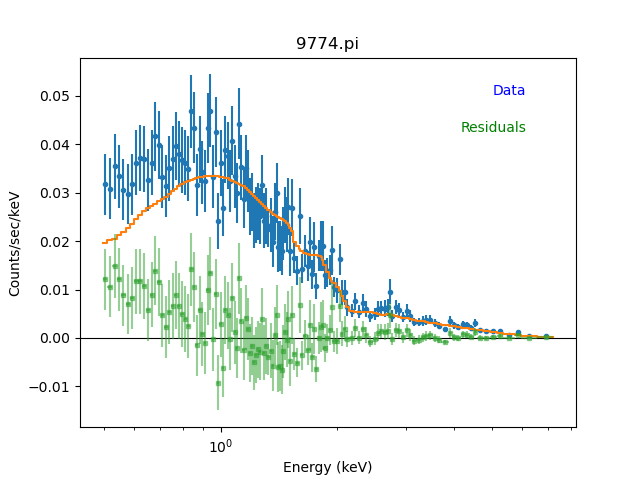### Overplotting residuals

The residuals are drawn with a small square symbol (rather than the default which is a small circular symbol) to help distinguish them from the data.

The labels have been added using "plot normalized" coordinates - where 0,0 is the bottom-left of the plot and 1,1 the top-right - rather than data coordinates, which is why the transform argument was used in the plt.text call.

You can overlay fits, as the matplotlib backend will automatically cycle colors:

```sherpa> plot_fit(1, alpha=0.5)
sherpa> plot_fit(2, alpha=0.5, overplot=True)
```

### Overplotting fits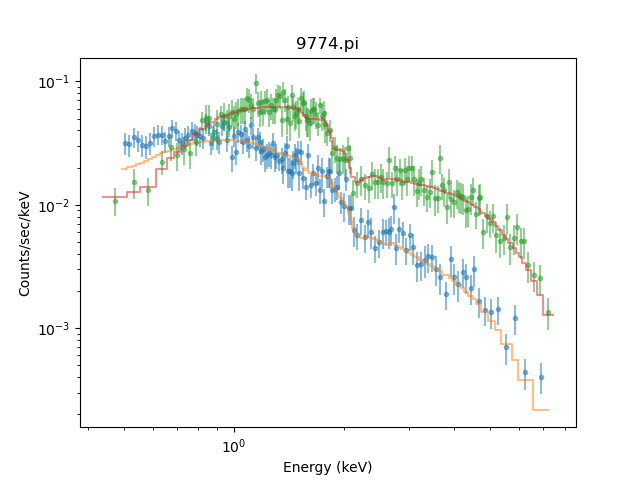### Overplotting fits

You can even combine data with different axes; for example in the following we add a second Y axis to the top plot to show how the ARF varies with energy:

```sherpa> plot_fit_ratio(1)
sherpa> plt.xlim(0.4, 8)
sherpa> fig = plt.gcf()
sherpa> plt.sca(fig.axes)
sherpa> ax2 = plt.twinx()
sherpa> plot_arf(1, overplot=True, color='gray', linestyle='dotted')
```

### Overplotting the ARF